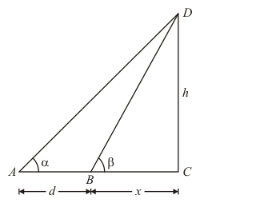# The angle of elevation of the top of a tower standing on a horizontal

Question:

The angle of elevation of the top of a tower standing on a horizontal plane from a point A is α. After walking a distance d towards the foot of the tower the angle of elevation is found to be β. The height of the tower is

(a) $\frac{d}{\cot \alpha+\cot \beta}$

(b) $\frac{d}{\cot \alpha-\cot \beta}$

(c) $\frac{d}{\tan \beta-\tan \alpha}$

(d) $\frac{d}{\tan \beta+\tan \alpha}$

Solution:

The given information can be represented with the help of a diagram as below.Here, CD = h is the height of the tower. Length of BC is taken as x.

In ΔACD,

$\tan A=\frac{C D}{A C}$

$\tan \alpha=\frac{h}{d+x}$

$h=(d+x) \tan \alpha$ .......(1)

In ΔBCD.

$\tan B=\frac{C D}{B C}$

$\tan \beta=\frac{h}{x}$

$x=h \cot \beta \quad \ldots(2)$

From (1) and (2),

$h=(d+h \cot \beta) \tan \alpha$

$h=d \tan \alpha+h \cot \beta \tan \alpha$

$h(1-\cot \beta \tan \alpha)=d \tan \alpha$

$h=\frac{d \tan \alpha}{(1-\cot \beta \tan \alpha)}=\frac{d}{\cot \alpha-\cot \beta}$

Hence the correct option is $b$